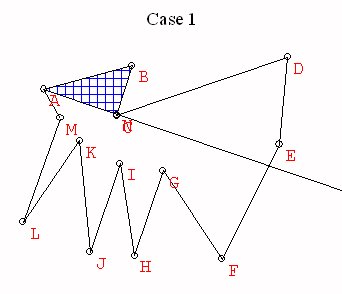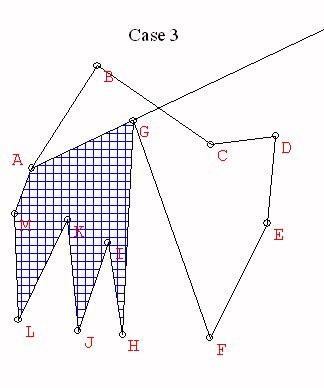The Triangulation of Any Polygonal Region in the Plane

The triangulation of any polygonal region in the plane is a key element in a proof of the equidecomposable polygon theorem.

Proposition:
Any region in the plane bounded by a closed polygon can be decomposed into the union of a finite number of closed triangular regions which intersect only on the boundaries.

The proof of this proposition examines a more careful characterization of the polygonal regions being considered. The key idea of the proof goes by induction on the number n = the number vertices = the number of sides in the polygon, as follows:

Proof:
Consider
n = the number vertices = the number of sides in the polygon
When n = 3 the result is trivial.

Suppose n> 3
and that for any polygon with k vertices/ sides, where k<n, the polygon can be triangulated. [An induction hypothesis.]
Now proceed to consider the vertices, v1,  v2, ..., vn ordered so that vi is adjacent to v(i+1) and vn is adjacent to v1.
Take a ray from v1 and rotate it from v1v2 so that it intersects the inside of the polygon. Continue to rotate until it meets another vertex.

Case 1.
This vertex is v3. Then consider the polygonal region Q1 = v1v3...vn which has n-1 vertices. By induction Q1 can be triangulated, so the original polygon is triangulated using the triangulation of Q1 and the triangle v1v2v3.Case 2. The vertex is v(n-1). Then consider the polygonal region Q2 = v1v2v3...vn-1 which has n-1 vertices. By induction Q2 can be triangulated, so the original polygon is triangulated using the triangulation of Q2 and the triangle v1vnv(n-1).Case 3. The vertex is vk with k different from 3 or n. Then consider the polygonal regions Q3 = v1v2...vk which has k vertices (k<n) and Q4 = v1vkv(k+1)...vn which has n-(k-2)<n vertices. By induction Q3 and Q4 can be triangulated, so the original polygon is triangulated using the triangulations of Q3 and Q4.This completes the argument that allows the use of induction to finish the proof of the proposition.

For more discussion of proofs of this proposition see Triangulations and arrangements, Two lectures by Godfried Toussaint, transcribed by Laura Anderson and Peter Yamamoto.